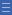## Featured resource

Home > Topdrawer > Mental computation > Activities > Parking ones and moving tens

# Parking ones and moving tens

Parking ones and moving tens

An important understanding of early strategic use of place value is what changes (ones, tens or both) in a given calculation. 'Parking ones' refers to the idea of leaving ones unchanged while working on tens.

Provide problems in situations where tens are added or subtracted from two-digit numbers that are not multiples of ten.

For example, 43 + 50 = ? or 96 – 30 = ?

Encourage students who initially skip count in tens (iterate by tens) to notice that only the tens digit changes.

Relate the answer to single digit addition and subtraction facts that they know.

For example, in 43 + 50 = 93 there are 5 tens added while 3 ones are 'parked'. You can watch the Tens Frame Parking video.

Problems that involve bridging 100 tend to be more difficult but are a sensible extension.

For example, 137 – 50 = 87 involves both parking ones and back-through-100 in tens.

Parking ones and moving tens can be practised using this empty number line applet on the New Zealand Maths website.

Yes

Yes

Name Class SectionYear 2: Solve simple addition and subtraction problems using a range of efficient mental and written strategies Infobox 3Source Infobox 3